# Extract Text from Cell – Excel & Google Sheets

This tutorial will demonstrate how to extract text from a cell in Excel and Google Sheets.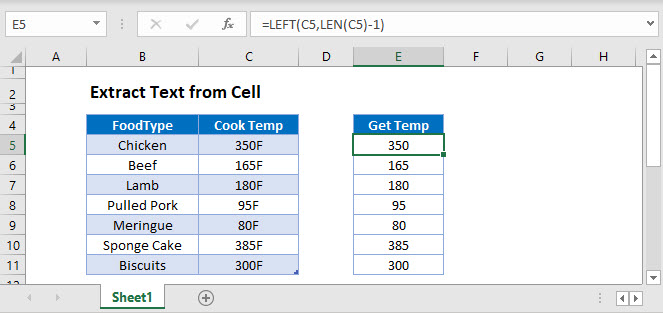## Extract Text from Left

You can extract text from the left side of a cell in Excel by using the LEFT Function. Simply supply the text, and enter the number of characters to return.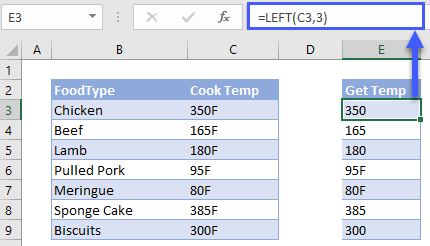However, this will only extract a fixed number of characters. You can see above some cook temperatures are correct extracted (ex. 300), but some are not (ex. 95F). To create a dynamic formula that will work in all scenarios are we can use the LEN Function, combined with the LEFT Function.

### LEN Function – Count Characters in a Cell

Use the LEN Function to count the number of characters in the cell:

``=LEN(C3)``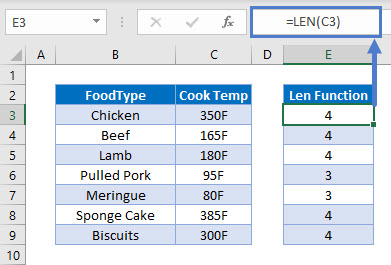### LEFT Function – Show Characters from the Left

Then, create a new LEFT Function that extracts a number of characters determined by the LEN Function created above.

``=LEFT(C3, E3-1)``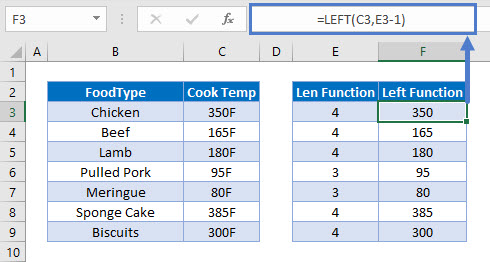Combining these functions looks like this:

``=LEFT(C3,LEN(C3)-1)``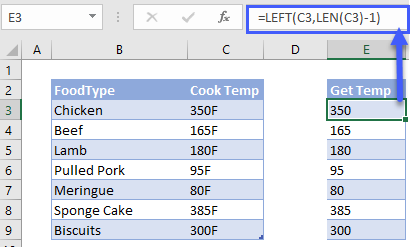### RIGHT and LEN Functions

Similarly, we can also extract characters from the right of a cell by using the RIGHT Function to return a certain number of characters from the right.

``=RIGHT(C3,LEN(C3)-n)``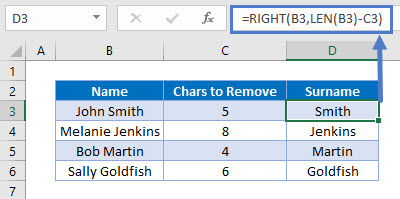## MID and SEARCH Functions

In the next section, we will use the SEARCH and MID functions to extract characters from the middle of a text string.

``=MID(B3,SEARCH(" ",B3)+1,999)``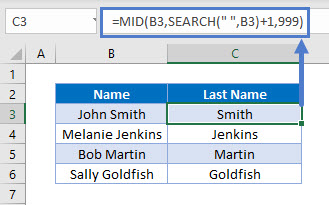### SEARCH Function

First, we use the SEARCH Function to find the position of the space (” “) between the first and last names.

``=SEARCH(" ", B3)``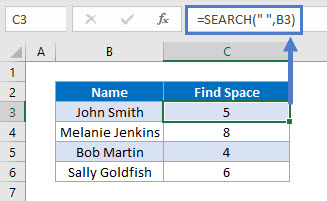### Easy Excel Automation### MID Function

Next, we use the MID Function to return all the characters after the space.

• We need to add 1 to the result of the previous formula so that we return the first character after the space.
• We use the large number 999 to return all characters.
``=MID(B3, C3+1, 999)``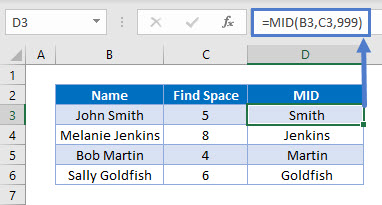Combining these 2 functions gives us the original formula for the last name.

``=MID(B3, SEARCH(B3, " ")+1, 999)``## Extract Text Before or After a Specific Character

You can also use the LEFT, RIGHT, LEN and SEARCH functions to extract the text before or after a specific character. In this case we will separate first and last names.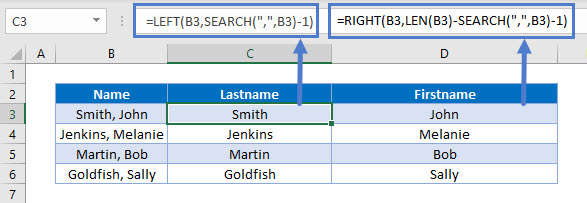### Extract Text Before Character

First, we can use the SEARCH Function to find the position of the comma in the text string.

``=SEARCH(",", B3)``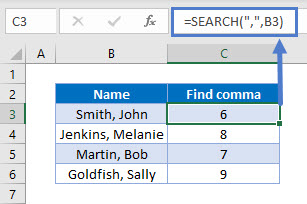Next, we can use the LEFT function to extract the text before the position of the comma.

• We need to subtract 1 from the position of the comma so not to include the comma in our result.
``=LEFT(B3, SEARCH(",",B3)-1)``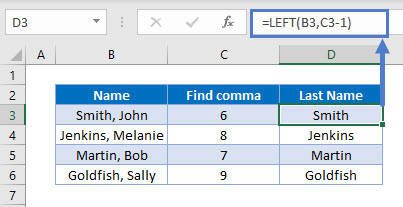Combining these 2 functions gives us the original formula for the last name.

### Extract Text After Character

``=RIGHT(B3,LEN(B3)-SEARCH(",",B3)-1)``In addition to using the SEARCH function once again, we also use the LEN function in conjunction with the RIGHT function to get extract text after a specific character.

The LEN Function is to get the length of the text in B3, while the SEARCH function is once again used to find the position of the comma.  We then use the RIGHT function to extract the characters after the comma in the text string.

## Extract Text From Middle of Text String

Next, we will discuss how to extract text from the middle of a text string

To extract text from the middle of a text string, we can use the RIGHT, SEARCH and LEN functions to get the text from the right side of the string, and then use the MID and LEN functions to get the text in the middle. We will also incorporate the TRIM function to trim any spaces on either side of the text string.

``````=RIGHT(B3,LEN(B3)-SEARCH(" ",B3)-LEN(TRIM(MID(B3,SEARCH(" ",B3,1)+1,
SEARCH(" ",B3,SEARCH(" ",B3,1)+1)-SEARCH(" ",B3,1))))-1)``````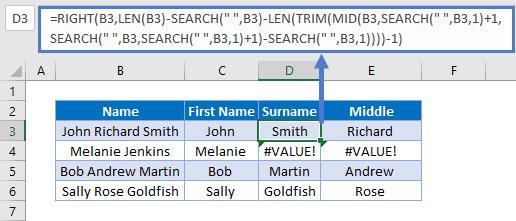This formula will only work if there is more than one space in the text string. If there is only one space, an error with #VALUE would be returned.

To solve this problem, for names without middle names or initials, we can use the original formula using the MID and SEARCH Functions.

``=MID(B3,SEARCH(" ",B3)+1,999))``

We can create a single formula that uses both techniques to handle all situations with the IFERROR Function. (The IFERROR Function performs a calculation. If that calculation results in an error, then another calculation is performed.)

``````=IFERROR(RIGHT(B3,LEN(B3)-SEARCH(" ",B3)-LEN(TRIM(MID(B3,SEARCH(" ",B3,1)+1,
SEARCH(" ",B3,SEARCH(" ",B3,1)+1)-SEARCH(" ",B3,1))))-1),MID(B3,SEARCH(" ",B3)+1,999))``````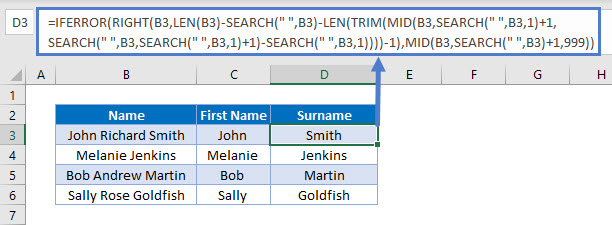We can then use the MID and LEN functions to obtain the middle name or initial.

``=MID(B3,LEN(C3)+1,LEN(B3)-LEN(C3&D3))``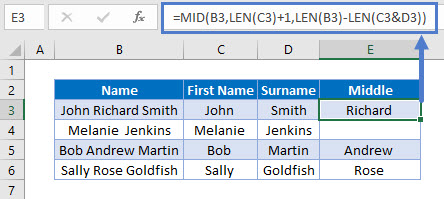## Extract Text From Cell in Google Sheets

All the examples above works the same way in Google Sheets.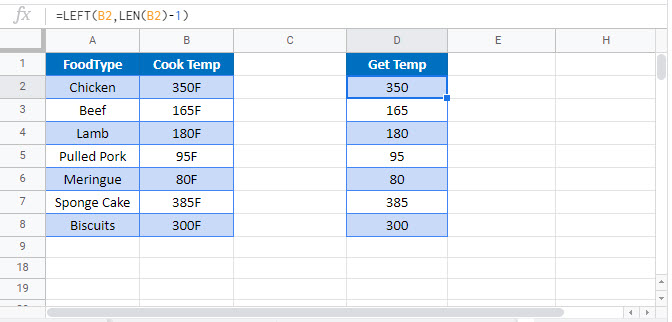### Excel Practice Worksheet

Practice Excel functions and formulas with our 100% free practice worksheets!